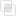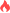python如何用函数创造字典

Python 函数 字典2021-06-25 15:23:181098浏览 · 0收藏 · 0评论1、使用dict()函数，通过其他映射（比如其他字典）或者键，值对的序列建立字典。

```dict1 = dict(a='a', b='b', t='t')     # 传入关键字
print(dict1)

dict2 = dict(zip(['one', 'two', 'three'], [1, 2, 3]))   # 映射函数方式来构造字典
print(dict2)

dict3 = dict([('one', 1), ('two', 2), ('three', 3)])    # 可迭代对象方式来构造字典
print(dict3)```

2、使用fromkeys()函数，只用来创建新字典，不负责保存。当通过一个字典来调用 fromkeys 方法时，如果需要后续使用一定记得给他复制给其他的变量。

```dict3 = dict.fromkeys(['name','age'])
print(dict3)

dict4 = dict.fromkeys(['name','age'],10)
print(dict4)```python元组的修改和删除python多线程的执行分析python字符串结合操作符的使用python中mainloop()方法的使用python设置的窗口位置python中del在垃圾回收的使用python线程中Condition的原理python线程事件Event的原理python建造者模式是什么python中Pylint是什么python中Pylint的信息类型python如何封装特性python继承的特性分析python多态的开闭原则php设置SESSION_ID的方法578

php中nts是什么308

JavaScript处理并行请求的方法192